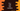# C++ program to add two numbers## C++ program to add two numbers:

In this post, I will show you how to add two numbers in a C++ program. This is a beginner level question and you will learn how we can read user inputs and how we can do arithmatic calculations in C++.

Our program will take two numbers as inputs from the user, adds them and print out the result on console.

so the program will:

• Ask the user to enter the first number. Read and store it in a variable.
• Ask the user to enter the second number. Read and store it in another variable.
• Add both numbers that user has entered. Store this result in another variable.
• Print the value in the result variable.

### C++ program:

Below is the complete C++ program :

``````#include<iostream>
using namespace std;

int main(){
int first, second, sum;

cout<<"Enter the first number : "<<endl;
cin >> first;

cout<<"Enter the second number : "<<endl;
cin>> second;

sum = first + second;

cout<<"Sum : "<<sum<<endl;
}``````

### Explanation:

Here,

• first, second and sum are three integer variables to store the first number, second number and sum of these numbers.
• Using cout, we are asking the user to enter the first number. Using cin, we are reading that number and storing that value in first.
• Similarly, we are reading and storing the second number in second.
• Both numbers are added using + and the sum is stored in sum.
• The last line prints the sum variable on console using cout.

### Sample output:

``````Enter the first number :
10
Enter the second number :
12
Sum : 22``````